## 一、从基本的使用去理解流

### 1、基本使用

``````import java.util.ArrayList;
import java.util.List;

import static java.util.Comparator.comparing;
import static java.util.stream.Collectors.toList;
public class StramMain {
private class Dash{
private int calories;
private String name;
public int getCalories() {
return calories;
}
public void setCalories(int calories) {
this.calories = calories;
}
public String getName() {
return name;
}
public void setName(String name) {
this.name = name;
}
}
public static void main(String[] args) {
.filter(d -> d.getCalories() < 400)//过滤低于400卡路里
.sorted(comparing(Dash::getCalories))//根据过滤之后进行排序
.map(Dash::getName)//对象映射成String类型
.collect(toList());//结束操作，转成list输出
System.out.println(lowColoricDishesName.toString());

}
}
``````

### 2、理解流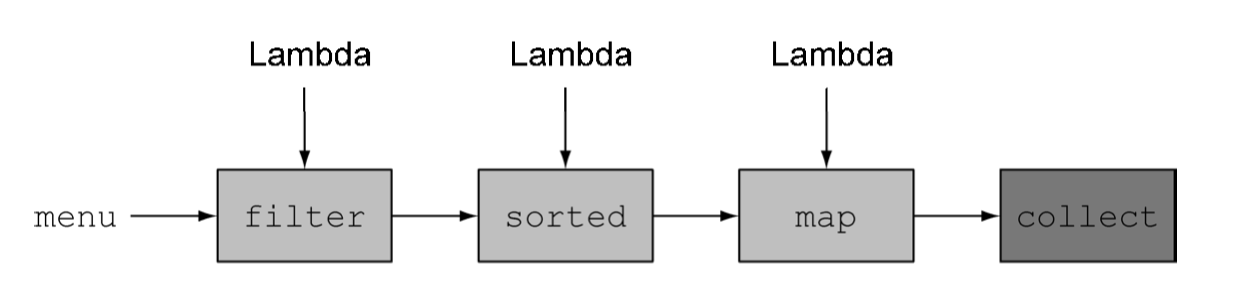• filter、sorted、map这些方法（操作），叫做中间操作
• collect这中方法（操作），叫做终端操作
• 重要的一点：除非流程线上触发一个终端操作，否则中间操作不会执行任何处理。所以说每个元素都只会被遍历一次！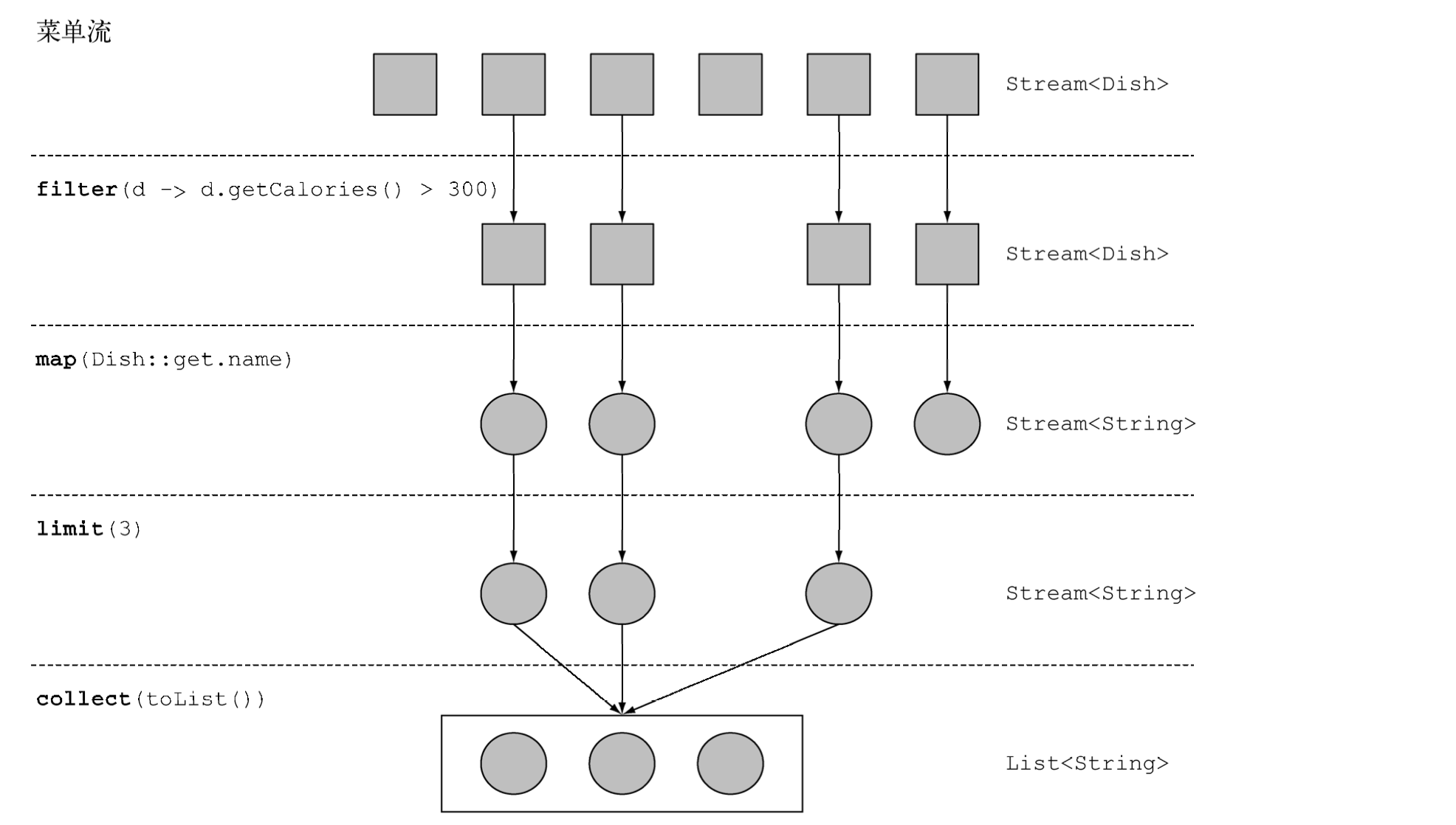• 可以进行短路：（如上图）在一个一个Dash经过流水线的过程中，到了limit(3)这里，发现，现在元素已经够了三个了，就不会进行下面元素的遍历了。这一点算是一种优化。这一点还可以运用到后面的anyMatch等终端操作中
• 只遍历一次：上面做过介绍，看似很长很长的流写法，其实对元素只内部遍历一次，甚至有时候不遍历，这个很牛逼
• 能够做内部优化：因为内部迭代，所以看似先后书写的流水线操作代码，其实不是按照书写顺序进行摆放的，内部会是有最优的顺序进行处理
• 能够并行去做迭代：这也是流的一大优势，如果使用并行流，内部迭代会自动分配不同任务到不同cpu上面，这种是我们自己写迭代器非常困难的

## 二、一些“风骚”的中间操作

• filter
• map
• limit

### 1、flatMap：“拍扁”操作

String[] words = {"jicheng","gufali"}

《Java 8 实战》里面尝试了两种方式，我觉得，很有助于我们思考这个拍扁操作的原理

#### 第一种尝试

``````public class StramMain {
public static void main(String[] args) {
String[] words = {"jicheng", "gufali"};
List<String[]> list = Arrays.stream(words)
.map(value -> value.split(""))
.distinct()
.collect(toList());
System.out.println(list);
}
}
``````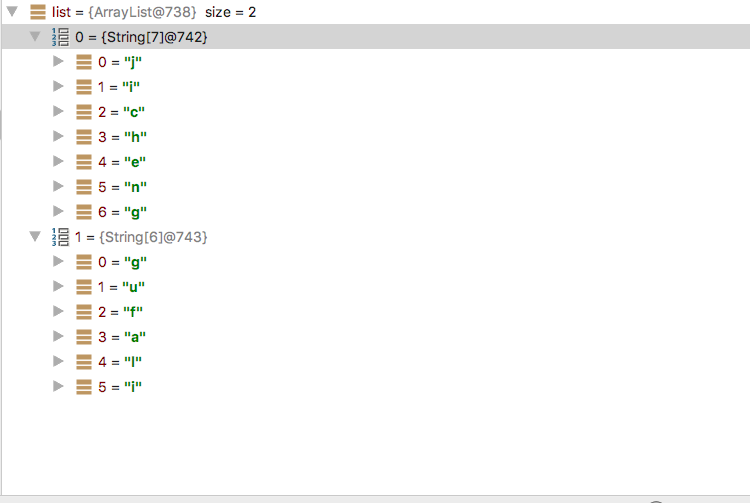#### 第二种尝试

``````import java.util.Arrays;
import java.util.List;
import java.util.stream.Stream;

import static java.util.stream.Collectors.toList;

public class StramMain {
public static void main(String[] args) {
List<String> words = Arrays.asList("jicheng", "gufali");
List<Stream<String>> collect = words.stream()
.map(value -> value.split(""))
.map(Arrays::stream)
.collect(toList());
System.out.println(collect);
}
}
``````
• 第一个map：将原始的流转成了`Stream<String[]>`类型
• 第二个map：分别将原String数组合并成了两个`Stream<String>`这样一个Stream流
• 最后：输出的就是`List<Stream<String>>`类型

#### 最终形态

``````import static java.util.stream.Collectors.toList;

public class StramMain {
public static void main(String[] args) {
List<String> words = Arrays.asList("jicheng", "gufali");
List<String> collect = words.stream()
.map(value -> value.split(""))
.flatMap(Arrays::stream)
.distinct()
.collect(toList());
System.out.println(collect);
// result:[j, i, c, h, e, n, g, u, f, a, l]
}
}
``````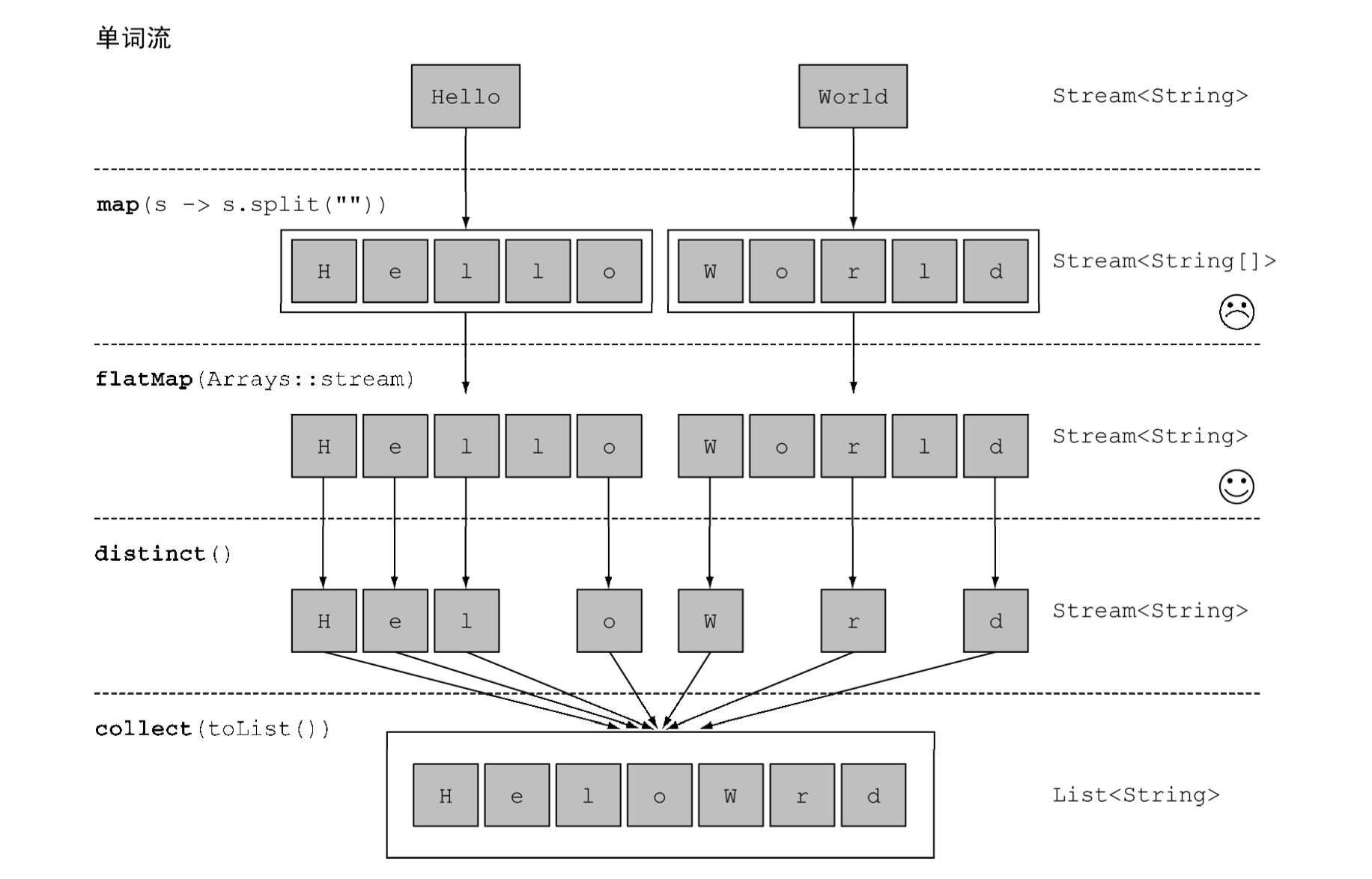### 2、findFirst/findAny：查找

``````Optional<Dish> dish =menu.stream()
.filter(Dish::isVegetarian)
.findAny();
boolean isPresent = dish.isPresent();
``````

• 返回的是一个Optional，接下来可以做几个处理：
• 直接使用isPresent方法，写一个if逻辑判断
• 或者直接在流的后面接`ifPresent(Consumer<T> block)`，如果值存在的话，会执行block
• findAny和findFirst的区别在于，findFirst返回集合中的第一个，findAny返回任意一个，对于使用并行流的时候，findFirst非常不好优化，有可能还是使用findAny

### 3、reduce：归约

``````Optional<T> reduce(BinaryOperator<T> accumulator);//①
<U> U reduce(U identity, BiFunction<U, ? super T, U> accumulator,
BinaryOperator<U> combiner);//②
T reduce(T identity, BinaryOperator<T> accumulator);//③
``````

#### a、详细解说一个的过程

``````public class StramMain {
public static void main(String[] args) {
List<Integer> numbers = Arrays.asList(1, 2, 3, 4, 5, 6, 7, 8, 9, 10);
Integer sum = numbers.stream().reduce(0, (a, b) -> a + b);
System.out.println(sum);
// result:55
}
}
``````

#### b、没有初始值的版本

``````public class StramMain {
public static void main(String[] args) {
List<Integer> numbers = Arrays.asList(1, 2, 3, 4, 5, 6, 7, 8, 9, 10);
Optional<Integer> reduce = numbers.stream().reduce((a, b) -> a + b);
System.out.println(reduce.get());
// result:55
}
}

``````

#### c、最大最小值

``````Optional<Integer> max = numbers.stream().reduce(Integer::max);
Optional<Integer> min = numbers.stream().reduce(Integer::min);
``````

``````public class StramMain {
public static void main(String[] args) {
List<Integer> numbers = Arrays.asList(1, 2, 3, 4, 5, 6, 7, 8, 9, 10);
Integer max = numbers.stream().reduce(Integer.MIN_VALUE, Integer::max);
Integer min = numbers.stream().reduce(Integer.MAX_VALUE, Integer::min);
System.out.println("max number:"+max+",min number:"+min);
// result:max number:10,min number:1
}
}
``````

### 4、IntStream/LongStream：数值流

Java 8引入了三个原始类型： IntStream 、 DoubleStream 和LongStream，分别将流中的元素特化为int、long和double，从而避免了暗含的装箱成本。每 个接口都带来了进行常用数值归约的新方法，比如对数值流求和的sum，找到最大元素的max。 此外还有在必要时再把它们转换回对象流的方法。要记住的是，这些特化的原因并不在于流的复杂性，而是装箱造成的复杂性——即类似int和Integer之间的效率差异。

#### a、映射到数值流

``````public class StramMain {
public static void main(String[] args) {

List<Integer> numbers = Arrays.asList(1,2,3,4,5);
int sum = numbers.stream()
.mapToInt(value -> value)
.sum();
System.out.println(sum);
// result:15
}
}
``````

#### b、转换回去

``````IntStream intStream = menu.stream().mapToInt(Dish::getCalories);
Stream<Integer> stream = intStream.boxed();
``````

#### c、最大最小值

``````public class StramMain {
public static void main(String[] args) {

List<Integer> numbers = Arrays.asList(1,2,3,4,5);
OptionalInt max = numbers.stream()
.mapToInt(value -> value)
.max();
OptionalInt min = numbers.stream()
.mapToInt(value -> value)
.min();
int maxValue = max.orElse(Integer.MAX_VALUE);//如果没有最大值默认给一个最大值
int minValue = min.orElse(Integer.MIN_VALUE);//如果没有最小值默认给一个最小值
}
}
``````

#### d、生成范围值

Java 8引入了两个可以用于IntStream和LongStream的静态方法，帮助生成这种范围： range和rangeClosed。这两个方法都是第一个参数接受起始值，第二个参数接受结束值。但 range是不包含结束值的，而rangeClosed则包含结束值：

``````IntStream evenNumbers = IntStream.rangeClosed(1, 100) .filter(n -> n % 2 == 0);//偶数流
System.out.println(evenNumbers.count());
``````

c、一个风骚的操作：求勾股数

``````public class StramMain {
public static void main(String[] args) {

Stream<double[]> pythagoreanTriples = IntStream.rangeClosed(1, 100)
.boxed()
.flatMap(a -> IntStream.rangeClosed(a, 100)
.mapToObj(b -> new double[]{a, b, Math.sqrt(a*a + b*b)})
.filter(t -> t % 1 == 0));
pythagoreanTriples.limit(3).forEach(value->{
System.out.println(value+","+value+","+value);
});
/**
* 结果：
* 3.0,4.0,5.0
* 5.0,12.0,13.0
* 6.0,8.0,10.0
*/
}
}

``````

### 5、Stream.iterate/Stream.generate：无限流

Stream API提供了两个静态方法来从函数生成流：Stream.iterate和Stream.generate。 这两个操作可以创建所谓的无限流：不像从固定集合创建的流那样有固定大小的流。

``````public class StramMain {
public static void main(String[] args) {

Stream.iterate(0, n -> n + 2)
.limit(10)//注释掉这一行，就会无限循环的生成下去
.forEach(System.out::println);
}
}
``````

``````public class StramMain {
public static void main(String[] args) {

Stream.generate(Math::random)
.limit(5)
.forEach(System.out::println);
}
}
``````

## 三、其实你不知道的终端操作

• 在终端操作也能完成的操作：汇总与规约
• 分组，分组在分组，分组再分组再分组。。。。。
• `List<Object>`变换成`Map<Object,Object>`，常用操作

### 1、在终端操作也能完成的操作：汇总与规约

#### a、基本示例

``````import com.alibaba.fastjson.JSON;

import java.util.Arrays;
import java.util.Comparator;
import java.util.IntSummaryStatistics;
import java.util.List;
import java.util.function.Function;

import static java.util.stream.Collectors.*;

public class StramMain {
public static void main(String[] args) {
List<Integer> intList = Arrays.asList(12, 23, 34, 54);
//计算一共有多少个值
Long collect = intList.stream().collect(counting());
//同上
Long sameWithCollect = intList.stream().count();
System.out.println("一共有多少个数字：" + collect);
//查找最大值
intList.stream()
.collect(maxBy(Comparator.comparing(Function.identity())))
.ifPresent(integer -> {
System.out.println("数字中的最大值：" + integer);
});

Integer integer = intList.stream()
.collect(summingInt(value -> value.intValue()));
System.out.println("所有数字的和是："+integer);
Double averageNumber = intList.stream()
.collect(averagingInt(value -> value.intValue()));
System.out.println("平均数是："+averageNumber);
IntSummaryStatistics intSummaryStatistics = intList.stream()
.collect(summarizingInt(value -> value.intValue()));
System.out.println("所有的归约汇总的结果对象是："
+ JSON.toJSONString(intSummaryStatistics));
/**
* result:
* 一共有多少个数字：4
* 数字中的最大值：54
* 所有数字的和是：123
* 平均数是：30.75
* 所有的归约汇总的结果对象是：{"average":30.75,"count":4,"max":54,"min":12,"sum":123}
*/

}
}
``````

#### b、连接字符串

`joining()`工厂方法返回的收集器会把对流中每一个对象应用toString方法得到的所有字符 串连接成一个字符串。另外，joining在内部使用了StringBuilder来把生成的字符串逐个追加起来。

``````import static java.util.stream.Collectors.*;
public class StramMain {
public static void main(String[] args) {
List<Integer> intList = Arrays.asList(12, 23, 34, 54);
String stringJoin = intList.stream()
.map(value -> value.toString())
.collect(joining(","));
System.out.println(stringJoin);
// result: 12,23,34,54
}
}
``````

#### c、广义的归约汇总

``````import java.util.Arrays;
import java.util.List;
import static java.util.stream.Collectors.reducing;
public class StramMain {
public static void main(String[] args) {
List<Integer> intList = Arrays.asList(12, 23, 34, 54);
Integer sumNumber = intList.stream()
//注意这里的reducing方法
.collect(reducing(0, value -> value.intValue(), Integer::sum));
System.out.println("求和："+sumNumber);
// result: 123
}
}
``````

• 第一个参数是归约操作的起始值，也是流中没有元素时的返回值，所以很显然对于数值和而言0是一个合适的值。
• 第二个参数是Function函数式接口，用于定位我们要返回的具体值类型
• 第三个参数是一个BinaryOperator，将两个项目累积成一个同类型的值，就是归约过程执行函数

### 2、分组，分组在分组，分组再分组再分组。。。。。

``````public final class Collectors {
...

//①
public static <T, K> Collector<T, ?, Map<K, List<T>>>
groupingBy(Function<? super T, ? extends K> classifier) {
...
}

//②
public static <T, K, A, D>
Collector<T, ?, Map<K, D>> groupingBy(Function<? super T, ? extends K> classifier,
Collector<? super T, A, D> downstream) {
...
}

...
}
``````
• 三个方法都返回同一个类型Collector
• ①方法是我们最常使用，最终使用collect方法能够返回`Map<K,List<O>>`类型
• 其中②方法就是实现多级分组的，可见第二个参数是一个Collector类型，我们可以再第二个参数地方调用①方法，如此递进下去
``````Map<Dish.Type, Map<CaloricLevel, List<Dish>>> dishesByTypeCaloricLevel = menu.stream()
.collect(groupingBy(Dish::getType, groupingBy(dish -> {
// 这里进行二次分组的实现函数
if (dish.getCalories() <= 400) return CaloricLevel.DIET;
else if (dish.getCalories() <= 700) return CaloricLevel.NORMAL;
else return CaloricLevel.FAT;
})));
``````

### 3、toMap的操作

``````public static <T, K, U>
Collector<T, ?, Map<K,U>> toMap(Function<? super T, ? extends K> keyMapper,
Function<? super T, ? extends U> valueMapper) {
}//①

public static <T, K, U>
Collector<T, ?, Map<K,U>> toMap(Function<? super T, ? extends K> keyMapper,
Function<? super T, ? extends U> valueMapper,
BinaryOperator<U> mergeFunction) {
}//②

public static <T, K, U, M extends Map<K, U>>
Collector<T, ?, M> toMap(Function<? super T, ? extends K> keyMapper,
Function<? super T, ? extends U> valueMapper,
BinaryOperator<U> mergeFunction,
Supplier<M> mapSupplier) {
BiConsumer<M, T> accumulator
= (map, element) -> map.merge(keyMapper.apply(element),
valueMapper.apply(element), mergeFunction);
return new CollectorImpl<>(mapSupplier, accumulator, mapMerger(mergeFunction), CH_ID);
}//③
``````

• ①方法能够直接将List映射成一个Map，第一个参数是key，第二个参数是value，key值重复会抛出IllegalStateException异常
• ②方法的第三个参数是避免如果出现了key值重复，如何选择的问题
• ③方法的第四个参数是决定具体返回是一个什么类型的map
``````Map<Integer,Person> idToPerson = persons.stream()
.collect(Collectors.toMap(Person::getId,Funtion.identity()));

Map<Integer,Person> idToPerson = persons.stream()
.collect(Collectors.toMap(Person::getId
,Funtion.identity()
,(existValue,newValue->existValue)));

TreeMap<Integer,Person> idToPerson = persons.stream()
.collect(Collectors.toMap(Person::getId
,Funtion.identity()
,(existValue,newValue->existValue)
,TreeMap::new));

``````### 心中的理想乡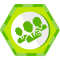06/16
0
0

06/16
0
0

06/28
0
0

06/11
0
0
【死磕Java并发】—– 死磕 Java 并发精品合集

【死磕 Java 并发】系列是 LZ 在 2017 年写的第一个死磕系列，一直没有做一个合集，这篇博客则是将整个系列做一个概览。 先来一个总览图： 【高清图，请关注“Java技术驿站”公众号，回复：脑...

chenssy
2018/07/22
0
0

Bootstrap(三)文本排版

ZeroBit
20分钟前
0
0

21分钟前
2
0

7月25日晚8点，线上直播，【AI中台——智能聊天机器人平台】，点击了解详情。 一、什么是AngularJS ? 1、AngularJS是一组用来开发web页面的框架、模板以及数据绑定和丰富UI的组件； 2、Angul...

25分钟前
2
0

26分钟前
0
0

linux服务器架构
26分钟前
1
0